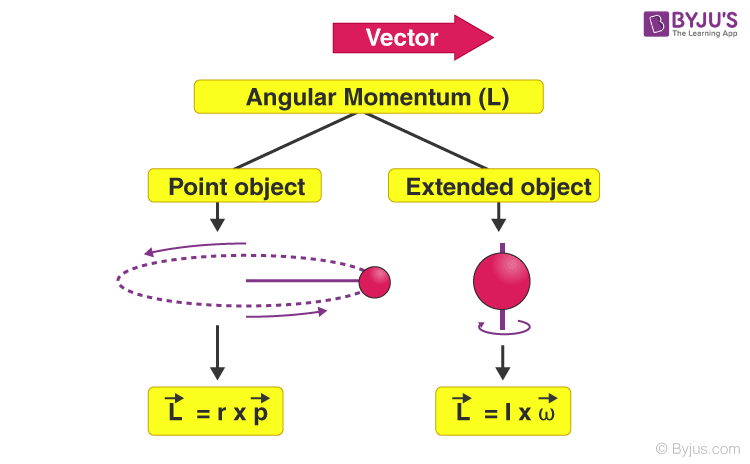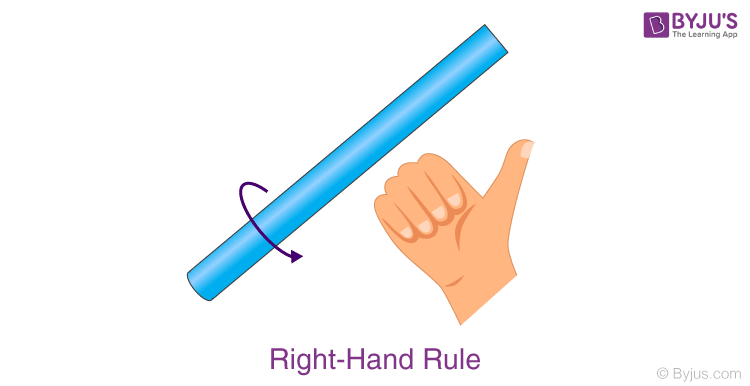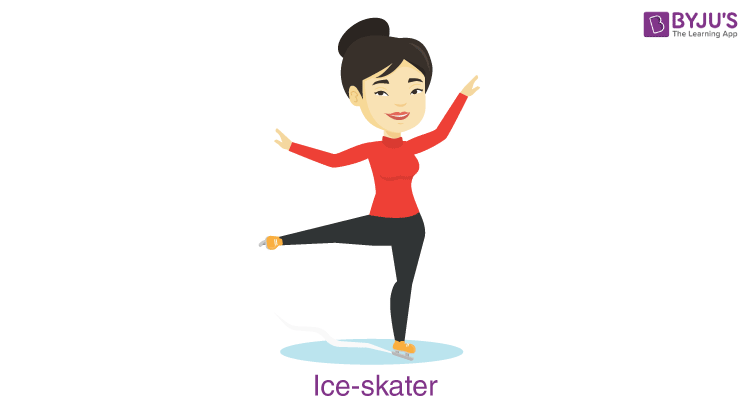# Angular Momentum

Momentum is the product of mass and the velocity of the object. Any object moving with mass possesses momentum. The only difference in angular momentum is that it deals with rotating or spinning objects. So is it the rotational equivalent of linear momentum?

## What is Angular Momentum?

If you try to get on a bicycle and balance without a kickstand, you will probably fall off. But once you start pedalling, these wheels pick up angular momentum. They are going to resist change, thereby making balancing gets easier.

Angular momentum is defined as:

The property of any rotating object given by moment of inertia times angular velocity.

It is the property of a rotating body given by the product of the moment of inertia and the angular velocity of the rotating object. It is a vector quantity, which implies that the direction is also considered here along with magnitude.

 Symbol The angular momentum is a vector quantity, denoted by $$\begin{array}{l}\vec{L}\end{array}$$ Units It is measured using SI base units: Kg.m2.s-1 Dimensional formula The dimensional formula is: [M][L]2[T]-1

You may also want to check out these topics given below!

## Angular Momentum Formula

Angular momentum can be experienced by an object in two situations. They are:

Point object: The object accelerating around a fixed point. For example, Earth revolving around the sun. Here the angular momentum is given by:

 $$\begin{array}{l}\vec{L}=\vec{r}\times \vec{p}\end{array}$$

Where,

• $$\begin{array}{l}\vec{L}\,is\,the\,angular\,velocity\end{array}$$
• r is the radius (distance between the object and the fixed point about which it revolves)
• $$\begin{array}{l}\vec{p}\,is\,the\,linear\,momentum.\end{array}$$

Extended object: The object, which is rotating about a fixed point. For example, Earth rotates about its axis. Here the angular momentum is given by:

 $$\begin{array}{l}\vec{L}=\vec{I}\times \vec{\omega }\end{array}$$

Where,

• $$\begin{array}{l}\vec{L}\,is\,the\,angular\,momentum.\end{array}$$
• I is the rotational inertia.
• $$\begin{array}{l} \vec{\omega }\,is\,the\,angular\,velocity.\end{array}$$### Recommended Video### Angular Momentum Quantum Number

Angular momentum quantum number is synonymous with Azimuthal quantum number or secondary quantum number. It is a quantum number of an atomic orbital that decides the angular momentum and describes the size and shape of the orbital. The typical value ranges from 0 to 1.

### Right-Hand RuleThe direction of angular momentum is given by the right-hand rule, which states that:

• If you position your right hand such that the fingers are in the direction of r.
• Then curl them around your palm such that they point towards the direction of Linear momentum(p).
• The outstretched thumb gives the direction of angular momentum(L).
 Similar Reading: Angular Velocity Angular Motion Angular Displacement Angular Acceleration

The video explains a problem based on the conservation of angular momentum. The solution to this physics merry-go-round problem is explained with the help of animations for your better understanding.## Examples of Angular Momentum

We knowingly or unknowingly come across this property in many instances. Some examples are explained below.

#### Ice-skater

When an ice-skater goes for a spin she starts off with her hands and legs far apart from the centre of her body. But when she needs more angular velocity to spin, she gets her hands and leg closer to her body. Hence, her angular momentum is conserved, and she spins faster.#### Gyroscope

A gyroscope uses the principle of angular momentum to maintain its orientation. It utilises a spinning wheel that has 3 degrees of freedom. When it is rotated at high speed it locks on to the orientation, and it won’t deviate from its orientation. This is useful in space applications where the attitude of a spacecraft is a really important factor to be controlled.

## Frequently Asked Questions – FAQs

Q1

### Calculate the angular momentum of a pulley of 2 kg, radius 0.1 m, rotating at a constant angular velocity of 4 rad/sec

Substitute the given values like m=2 kg and r=0.1 m in I=(1/2)mr² (formula of the moment of inertia) we get I= 0.01 kg.m2
Angular momentum is given by L=Iω, thus, substituting the values we get L=0.04 kg.m².s-¹.
Q2

### Give the expression for Angular momentum.

$$\begin{array}{l}\vec{L}=\vec{I} \times \vec{\omega }\end{array}$$
or
$$\begin{array}{l}\vec{L}=\vec{r} \times \vec{p}\end{array}$$
Q3

### For an isolated rotating body, how are angular velocity and radius related?

For an isolated rotating body, angular velocity is inversely proportional to the radius.

Q4

### Write the dimensional formula for Angular momentum.

The dimensional formula is ML2T-1
Q5

### When an ice-skater goes for a spin, what happens to her spinning speed when she stretches her hands?

Spinning speed reduces.
Q6

### How can an ice-skater increase his/her spinning speed?

By bringing hands closer, thus reducing the radius increases the angular velocity.
Q7

### If the moment of inertia of an isolated system is halved. What happens to its angular velocity?

Angular velocity will be doubled.
Q8

### Calculate the angular moment of the object. When an object with the moment of inertia I = 5 kg.m² is made to rotate 1 rad/sec speed.

Substituting the given value in formula L=Iω we get L=5 kg.m2.s-1.Stay tuned with BYJU’S for more such interesting articles. Also, download BYJU’S – The Learning App for loads of interactive, engaging Physics videos with unlimited academic assistance.

Test your knowledge on Angular Momentum